## Tuesday, December 15, 2015

### NCDF(S, 2, 2, L, 3): Example 3: S = [1, 1, 1, 1, 4, 4, 4, 4]

Problem

This post is a detailed example of computing individual elements of NCDF(S, 2, 2, L, 3) at each of the three scales on S = [1, 1, 1, 1, 4, 4, 4, 4]. NCDF(S, 2, 2, L, J) notation is defined here. This notation is based on the notation introduced in Chapters 3 and 4 in "Ripples in Mathematics" by A. Jensen & A. la Cour-Harbo.

NCDF(S, 2, 2, 1, 3): Scale L = 1

Figures 1 and 2 show the formulas for the elements of S and D at scale L = 1. Figure 3 shows an example of computing the first scale of the signal S = [1, 1, 1, 1, 4, 4, 4, 4].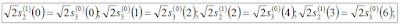Figure 1. Formulas for elements of S at scale L = 1Figure 2. Formulas for elements of D at scale L = 1Figure 3. Computing elements of S and D at scale L = 1

NCDF(S, 2, 2, 2, 3): Scale L = 2

Figure 4 shows the individual formulas for S and D at scale L = 2. Figure 5 shows the computation of individual elements of S and D of the sample S =  [1, 1, 1, 1, 4, 4, 4, 4] at scale L = 2.Figure 4. Formulas for elements of S and D at scale L = 2Figure 5. Computing elements of S and D at scale L = 2

NCDF(S, 2, 2, 3, 3): Scale L = 3

Figure 6 shows the individual formulas for S and D at scale L = 3. Figure 7 shows the computation of individual elements of S and D of the sample S =  [1, 1, 1, 1, 4, 4, 4, 4] at scale L = 3.Figure 6. Formulas for elements of S and D at scale L = 3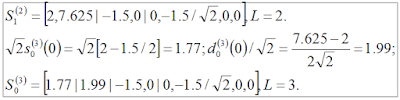Figure 7. Computing elements of S and D at scale L = 3

Summary of NCDF(S, 2, 2, 3, 3) at All Three Scales

Figure 8 summarizes the three scales of NCDF(S, 2, 2, 3, 3), where S =  [1, 1, 1, 1, 4, 4, 4, 4].Figure 8. Summary of three scales of NCDF(S, 2, 2, 3, 3) on S = [1, 1, 1, 1, 4, 4, 4, 4]

### SCDF(S, 2, 2, L, 3): Example 3: S = [1, 1, 1, 1, 4, 4, 4, 4]

Problem

This post is a detailed example of computing individual elements of SCDF(S, 2, 2, L, 3) at each of the three scales on S = [1, 1, 1, 1, 4, 4, 4, 4]. SCDF(S, 2, 2, L, J) notation is defined here. This notation is based on the notation introduced in Chapters 3 and 4 in "Ripples in Mathematics" by A. Jensen & A. la Cour-Harbo.

SCDF(S, 2, 2, 1, 3): Scale L = 1

Figures 1 and 2 show the formulas for the elements of S and D at scale L = 1. Figure 3 shows an example of computing the first scale of the signal S = [1, 1, 1, 1, 4, 4, 4, 4].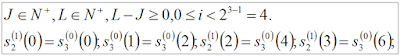Figure 1. Formulas for individual elements of S at scale L = 1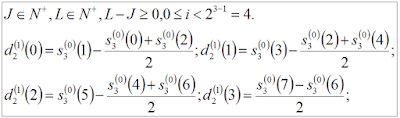Figure 2. Formulas for individual elements of D at scale L = 1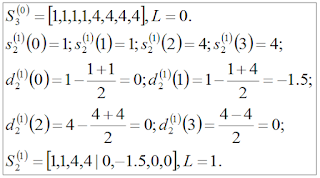Figure 3. Computing elements of S and D at scale L = 1

SCDF(S, 2, 2, 2, 3): Scale L = 2

Figure 4 shows the individual formulas for S and D at scale L = 2. Figure 5 shows the computation of individual elements of S and D of the sample S = [1, 1, 1, 1, 4, 4, 4, 4] at scale L = 2.Figure 4. Formulas for individual elements of S and D at scale L = 2Figure 5. Computing elements of S and D at scale L = 2

SCDF(S, 2, 2, 3, 3): Scale L = 3

Figure 6 shows the individual formulas for S and D at scale L = 3. Figure 7 shows the computation of individual elements of S and D of the sampleS = [1, 1, 1, 1, 4, 4, 4, 4] at scale L = 3.Figure 6. Formulas for individual elements of S and D at scale L = 3Figure 7. Computing elements of S and D at scale L = 3

Summary of SCDF(S, 2, 2, 3, 3) at All Three Scales

Figure 8 summarizes the three scales of SCDF(S, 2, 2, 3, 3), where S =  S = [1, 1, 1, 1, 4, 4, 4, 4] .Figure 8. Summary of SCDF(S, 2, 2, 3, 3) for three scales on S = [1, 1, 1, 1, 4, 4, 4, 4]

### NCDF(S, 2, 2, L, 3): Example 2: S = [8, 7, 6, 5, 4, 3, 2, 1]

Problem

This post is a detailed example of computing individual elements of NCDF(S, 2, 2, L, 3) at each of the three scales on S = [8, 7, 6, 5, 4, 3, 2, 1]. NCDF(S, 2, 2, L, J) notation is defined here. This notation is based on the notation introduced in Chapters 3 and 4 in "Ripples in Mathematics" by A. Jensen & A. la Cour-Harbo.

NCDF(S, 2, 2, 1, 3): Scale L = 1

Figures 1 and 2 show the formulas for the elements of S and D at scale L = 1. Figure 3 shows an example of computing the first scale of the signal S = [8, 7, 6, 5, 4, 3, 2, 1].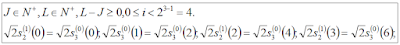Figure 1. Formulas for individual elements of S at scale L = 1Figure 2. Formulas for individual elements of D at scale L = 1Figure 3. Computing individual elements of S and D at scale L = 1

NCDF(S, 2, 2, 2, 3): Scale L = 2

Figure 4 shows the individual formulas for S and D at scale L = 2. Figure 5 shows the computation of individual elements of S and D of the sample S = [8, 7, 6, 5, 4, 3, 2, 1]. at scale L = 2.Figure 4. Formulas for individual elements of S and D at scale L = 2Figure 5. Computing individual elements of S and D at scale L = 2

NCDF(S, 2, 2, 3, 3): Scale L = 3

Figure 6 shows the individual formulas for S and D at scale L = 3. Figure 7 shows the computation of individual elements of S and D of the sample S = [8, 7, 6, 5, 4, 3, 2, 1]. at scale L = 3.Figure 6. Formulas for individual elements of S and D at scale L = 3Figure 7. Computing elements of S and D at scale L = 3

Summary of NCDF(S, 2, 2, 3, 3) at All Three Scales

Figure 8 summarizes the three scales of NCDF(S, 2, 2, 3, 3), where S = [8, 7, 6, 5, 4, 3, 2, 1].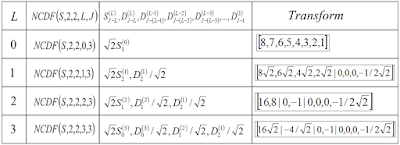Figure 8. Summary of NCDF(S, 2, 2, 3, 3) at all three scales on S = [8, 7, 6, 5, 4, 3, 2, 1]

### SCDF(S, 2, 2, L, 3): Example 2: S = [8, 7, 6, 5, 4, 3, 2, 1]

Problem

This post is a detailed example of computing individual elements of SCDF(S, 2, 2, L, 3) at each of the three scales on S = [8, 7, 6, 5, 4, 3, 2, 1]. SCDF(S, 2, 2, L, J) notation is defined here. This notation is based on the notation introduced in Chapters 3 and 4 in "Ripples in Mathematics" by A. Jensen & A. la Cour-Harbo.

SCDF(S, 2, 2, 1, 3): Scale L = 1

Figures 1 and 2 show the formulas for the elements of S and D at scale L = 1. Figure 3 shows an example of computing the first scale of the signal S = [8, 7, 6, 5, 4, 3, 2, 1].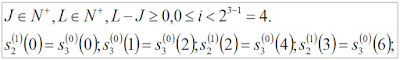Figure 1. Formulas for elements of S at scale L = 1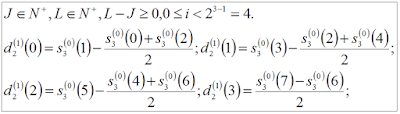Figure 2. Formulas for elements of D at scale L = 1Figure 3. Computing elements of S and D at scale L = 1

SCDF(S, 2, 2, 2, 3): Scale L = 2

Figure 4 shows the individual formulas for S and D at scale L = 2. Figure 5 shows the computation of individual elements of S and D of the sample [8, 7, 6, 5, 4, 3, 2, 1] at scale L = 2.Figure 4. Formulas for elements of S and D at scale L = 2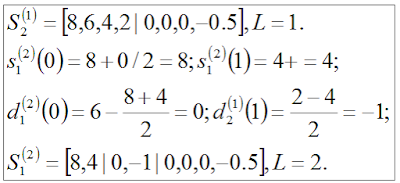Figure 5. Computing elements of S and D at scale L = 2

SCDF(S, 2, 2, 3, 3): Scale L = 3

Figure 6 shows the individual formulas for S and D at scale L = 3. Figure 7 shows the computation of individual elements of S and D of the sample [8, 7, 6, 5, 4, 3, 2, 1] at scale L = 3.Figure 6. Formulas for individual elements of S and D at scale L = 3Figure 7. Computing elements of S and D at scale L = 3

Summary of SCDF(S, 2, 2, 3, 3) at All Three Scales

Figure 8 summarizes the three scales of SCDF(S, 2, 2, 3, 3), where S =  [8, 7, 6, 5, 4, 3, 2, 1] .Figure 8. Computing three scales of SCDF(S, 2, 2, 3, 3) for S =  [8, 7, 6, 5, 4, 3, 2, 1]

### NCDF(S, 2, 2, L, 3): Example 1: S = [1, 2, 3, 4, 5, 6, 7, 8]

Problem

This post is a detailed example of computing individual elements of NCDF(S, 2, 2, L, 3) at each of the three scales on S = [1, 2, 3, 4, 5, 6, 7, 8]. NCDF(S, 2, 2, L, J) notation is defined here. This notation is based on the notation introduced in Chapters 3 and 4 in "Ripples in Mathematics" by A. Jensen & A. la Cour-Harbo.

NCDF(S, 2, 2, 1, 3): Scale L = 1

Figures 1 and 2 show the formulas for the elements of S and D at scale L = 1. Figure 3 shows an example of computing the first scale of the signal S = [1, 2, 3, 4, 5, 6, 7, 8].Figure 1. Formulas for individual elements of S at scale L = 1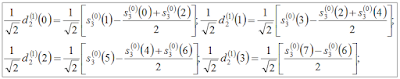Figure 2. Formulas for individual elements of D at scale L = 1Figure 3. Computing elements of S and D at scale L = 1

NCDF(S, 2, 2, 2, 3): Scale L = 2

Figure 4 shows the individual formulas for S and D at scale L = 2. Figure 5 shows the computation of individual elements of S and D of the sample [1, 2, 3, 4, 5, 6, 7, 8] at scale L = 2.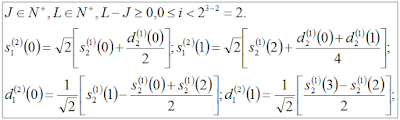Figure 4. Formulas for individual elements of S and D at scale L = 2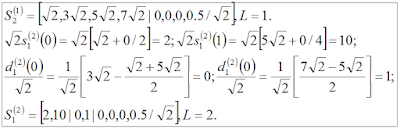Figure 5. Computing elements of S and D at scale L = 2

NCDF(S, 2, 2, 3, 3): Scale L = 3

Figure 6 shows the individual formulas for S and D at scale L = 3. Figure 7 shows the computation of individual elements of S and D of the sample [1, 2, 3, 4, 5, 6, 7, 8] at scale L = 3.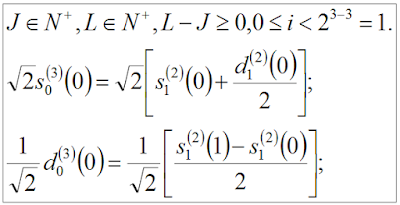Figure 6. Formulas for individual elements of S and D at scale L = 3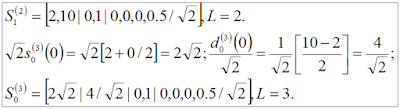Figure 7. Computing elements of S and D at scale L = 3

Summary of NCDF(S, 2, 2, 3, 3) at All Three Scales

Figure 8 summarizes the three scales of NCDF(S, 2, 2, 3, 3), where S = [1, 2, 3, 4, 5, 6, 7, 8].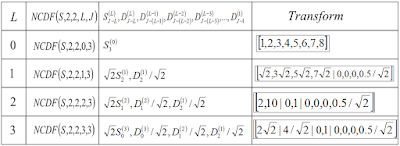Figure 8. Summary of NCDF(S, 2, 2, 3, 3) at all three scales

### SCDF(S, 2, 2, L, 3): Example 1: S = [1, 2, 3, 4, 5, 6, 7, 8]

Problem

This post is a detailed example of computing individual elements of SCDF(S, 2, 2, L, 3) at each of the three scales on S = [1, 2, 3, 4, 5, 6, 7, 8]. SCDF(S, 2, 2, L, J) notation is defined here. This notation is based on the notation introduced in Chapters 3 and 4 in "Ripples in Mathematics" by A. Jensen & A. la Cour-Harbo.

SCDF(S, 2, 2, 1, 3): Scale L = 1

Figures 1 and 2 show the formulas for the elements of S and D at scale L = 1. Figure 3 shows an example of computing the first scale of the signal S = [1, 2, 3, 4, 5, 6, 7, 8].Figure 1. Formulas for individual elements of S at scale L = 1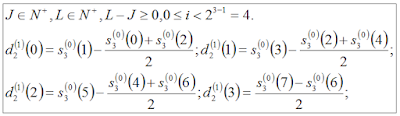Figure 2. Formulas for individual elements of D at scale L = 1Figure 3. Computing individual elements of S and D at scale L = 1

SCDF(S, 2, 2, 2, 3): Scale L = 2

Figure 4 shows the individual formulas for S and D at scale L = 2. Figure 5 shows the computation of individual elements of S and D of the sample [1, 2, 3, 4, 5, 6, 7, 8] at scale L = 2.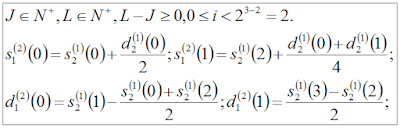Figure 4. Formulas for individual elements of S and D at scale L = 2Figure 5. Computing individual elements of S and D of [1, 2, 3, 4, 5, 6, 7, 8] at scale L = 2

SCDF(S, 2, 2, 3, 3): Scale L = 3

Figure 6 shows the individual formulas for S and D at scale L = 3. Figure 8 shows the computation of individual elements of S and D of the sample [1, 2, 3, 4, 5, 6, 7, 8] at scale L = 3.Figure 6. Formulas for individual elements of S and D at scale L = 3Figure 7. Computing individual elements of S and D of [1, 2, 3, 4, 5, 6, 7, 8] at scale L = 3

Summary of SCDF(S, 2, 2, 3, 3) at All Three Scales

Figure 8 summarizes the three scales of SCDF(S, 2, 2, 3, 3), where S = [1, 2, 3, 4, 5, 6, 7, 8].Figure 8. Summary of SCDF(S, 2, 2, 3, 3) Example 1

## Monday, December 14, 2015

### Three Scales of SCDF(S, 2, 2, L, 3) and NCDF(S, 2, 2, L, 3) and Formulas for Individual Elements at Each Scale: Conceptual Note 4 on Ripples in Mathematics

Introduction

This is my conceptual note 4 on Chapters 3 and 4 in "Ripples in Mathematics" by A. Jensen & A. la Cour-Harbo. This note continues to discuss the notation SCDF(S, 2, 2, L, J) and NCDF(S, 2, 2, L, J) introduced in conceptual note 3. Recall that in  SCDF(S, 2, 2, L, J) the first letter stands for standard whereas in NCDF(S, 2, 2, L, J) the first letter stands for normalized. Jensen and la Cour-Harbo give the normalization formulas in equations 3.40 and 3.41 on p. 23, where the signal samples, i.e., the elements of S, are normalized by sqrt(2) and the elements of D are normalized by 1/sqrt(2). In this note, I will derive the formulas for the three iterations for SCDF(S, 2, 2, L, 3) and NCDF(S, 2, 2, L, 3) and derive the formulas for the individual elements for L = 1, 2, and 3. These manual derivations have helped me verify my implementation of CDF(2, 2) on shorter signals.

Three Scales of SCDF(S,2,2,L,J) and NCDF(S, 2, 2, L, J)

Figures 1 and 2 show how three scales of SCDF(S, 2, 2, L, 3) and NCDF(S, 2, 2, L, 3) are computed, respectively. In Figure 2, if the images for NCDF(S, 2, 2, L, 3) appear blurry, even when enhanced, each element of S, at each of the three scales, is multiplied by sqrt(2) and each element of D, at each scale of the three scales, is divided by sqrt(2), per the normalization formulas on p. 23 in "Ripples in Mathematics."Figure 1. Three Scales of SCDF(2, 2, L, 3).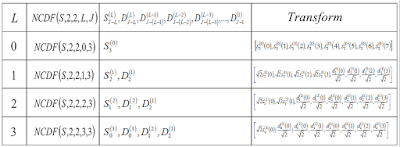Figure 2. Three Scales of NCDF(2, 2, L, 3)

Formulas for Individual Elements at Each Scale of SCDF(S, 2, 2, L, 3)

Figure 3 - 7 define formulas for the individual elements of S and D at each of the three scales of SCDF(S, 2, 2, L, 3).Figure 3. Formulas for the S elements at L = 1Figure 4. Formulas for the D elements at L = 1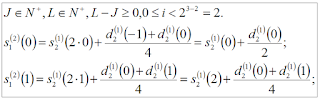Figure 5. Formulas for the S elements at L = 2Figure 6. Formulas for the D elements at L = 2Figure 7. Formulas for the S and D elements at L = 3

Formulas for Individual Elements at Each Scale of NCDF(S, 2, 2, L, 3)

Figures 8 - 12 state the formulas for the individual elements of S and D at each scale of NCDF(S, 2, 2, L, 3).Figure 8. Formulas for the S elements at L = 1Figure 9. Formulas for the D elements at L = 1Figure 10. Formulas for the S elements at L = 2Figure 11. Formulas for the D elements at L = 2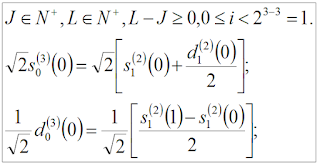Figure 12. Formulas for the S and D elements at L = 3